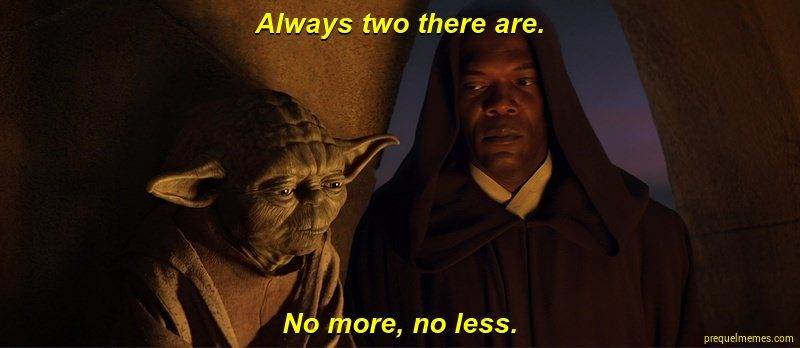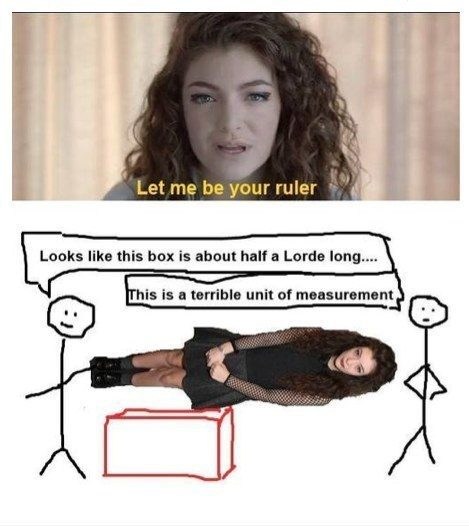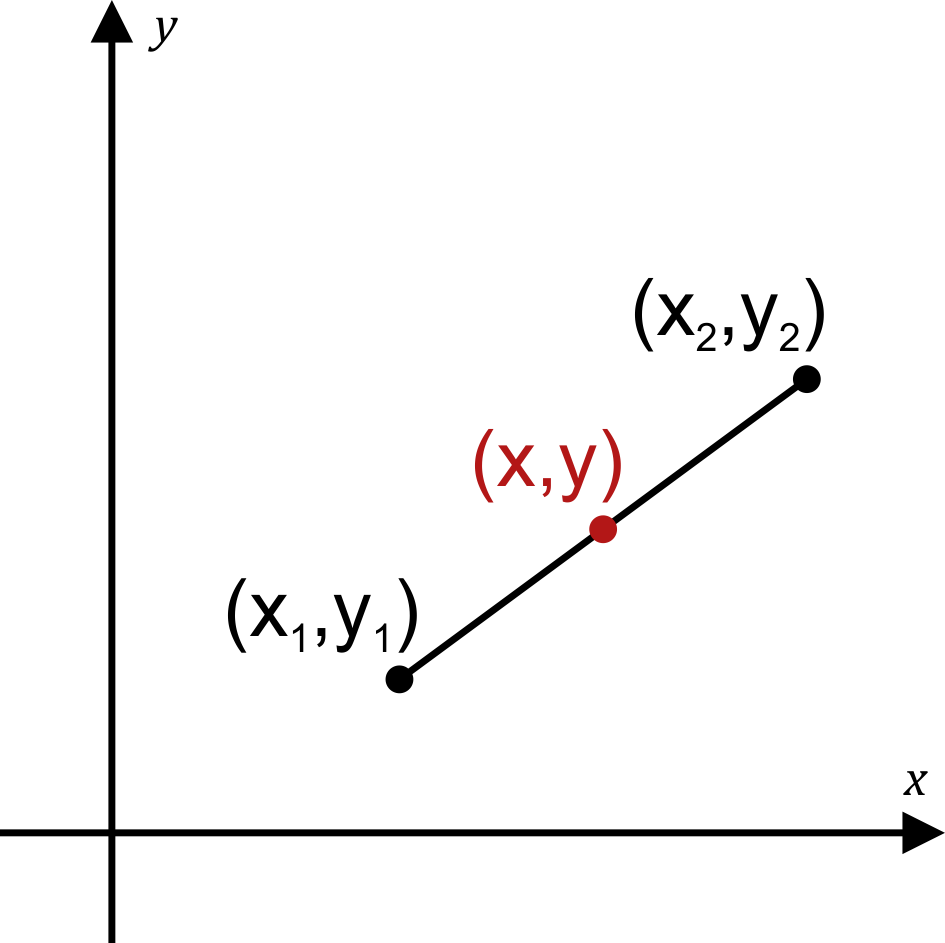# Endpoint Calculator

Created by Maciej Kowalski, PhD candidate
Reviewed by Bogna Szyk and Jack Bowater
Last updated: Sep 26, 2022

Welcome to Omni's endpoint calculator, where we'll learn how to find the endpoint of a line segment if we know its other end and its midpoint. As you might have guessed, this topic is connected to computing the midpoint, which is the reason why the endpoint formula is quite similar to that of the midpoint calculator. But, before we go into details, we'll slowly go through the endpoint definition in geometry to better understand what we're dealing with here.

So, sit back, brew yourself a cup of tea for the journey, and let's get to it!

## Endpoint definition in geometry

Colloquially speaking, an endpoint is a point that lies at the end. We're sure that this statement was as much of a shock to you as it was to us when we heard it first. But, on the other hand, eggplant doesn't taste like eggs at all, so you can never be too sure when guessing the meaning of a word, can you?

However, there are times, like when you're splitting a pizza for several people, where you have to be a bit more precise, and who else could we turn to for that if not mathematicians?

In its simplest form, the endpoint definition in geometry focuses on line segments, i.e., straight lines connecting two points. Yup, you guessed it – these points are called endpoints. Note that according to this definition, each segment has two endpoints (unless it's the degenerate case where they are the same point, i.e., the interval is a single point).For simplicity and calculations, we'll call one of them the starting point (as is done in the endpoint calculator). Keep in mind, however, that the beginning can just as well be the end if you look at it from the other side.

Now, that sounded creepily philosophical, don't you think? But let's leave the "Who are we and where do we go? questions for when we can't fall asleep. We should focus on the segments we've mentioned and how to find the endpoints.

## How to find the endpoint?

In order to get the endpoint, we need to have some point of reference to begin with. In other words, since we're dealing with a line segment and one of its components, we need to know what the rest of it looks like.

The simplest and most common situation is where we're missing the endpoint while we know the starting point and the midpoint. The latter is simply, as the name suggests, the point marking the middle of the segment. This is all that we need to find the endpoint; after all, it must lie at the other end of the midpoint from the starting point and be the same distance away.

Therefore, intuitively, we can already geometrically describe how to find the endpoint.

1. Given the starting point, $A$, and the midpoint, $B$, draw the line segment that connects the two.
2. Draw a line going farther from $B$ away from $A$ to God-knows-where.
3. Measure the distance from $A$ to $B$ and mark the same distance from $B$ going the other way.
4. Proceed to do a victory dance.

However, there are people (and we're not suggesting that we are those people) that don't really enjoy drawing lines that much. After all, you need a ruler for that, and Lorde is hard to come by... (Yes, that was a terrible joke, and we bow our heads in shame. But with a slight snigger, nonetheless.)

🔎 Instead of drawing lines, you can use our distance calculator for two given points.Anyway, for people who prefer numbers and calculations (and we might actually be suggesting that we are those people), we'll focus on how to find the endpoint algebraically in . Please, don't be afraid of the word 'algebraically' – in a second, you'll see how it translates to "easily and effortlessly" – the very motto of our missing endpoint calculator.

## Endpoint formula

In coordinate geometry, we handle objects that are embedded in what we call Euclidean space. It's not too important right now to understand its mathematical definition, but, for our purposes, it's enough to know that this means that in such spaces, points, say, $A$ or $B$, have two coordinates: $A = (x_1, y_1)$ and $B = (x_2, y_2)$.

The numbers $x_1$ and $x_2$ mark the position of the points with respect to the horizontal axis (usually denoted with $x$'s), while $y_1$ and $y_2$ are used for the vertical axis (most often denoted with $y$'s). Together, such a pair of numbers $(x_1, y_1)$ defines a point in the space. What is more, the coordinates help us analyze more complicated objects in our Euclidean space. For instance, they appear in the endpoint formula.

Say that you have a line segment going from $A = (x_1, y_1)$ to... well, we don't yet know. We will now explain how to find the endpoint $B = (x_2, y_2)$ if we know the midpoint $M = (x, y)$.

From the definition of a midpoint, we know that the distance from $A$ to $M$ must be the same as that from $M$ to $B$. It's just that B is on the other side. This means that to find $B$, it is enough to "move" $M$ along the line going through $A$ and $M$ by the same length as that of the segment $AM$. Or, if you'd like to sound fancy, by the vector $AM$.

In other words, we have:

$x_2 = x + (x - x_1) = 2x - x_1$, and

$y_2 = y + (y - y_1) = 2y - y_1$.

To sum it all up, if you like having all the information you need in one paragraph, then there it is.

💡 The endpoint of a line segment going from $A = (x_1, y_1)$ to a midpoint at $M = (x, y)$ is the point $B = (2x - x_1, 2y - y_1)$.

Note that above we've mentioned the line going through A and M. Such lines are quite helpful when learning to find the endpoint or the midpoint. After all, the segment AB is contained on that line. If your exercise or problem requires more information about them, check out Omni's and find the one that suits your needs!

Phew, that was a long time spent on theory! How about we leave this technical mumbo-jumbo and see a numerical example? Time is money, after all – or at least that's what the time value of money formula tells us!

## Example: using the missing endpoint calculator

Say that four months ago, you began posting videos on YouTube. Nothing fancy, just that are traditional to your region. It started as a hobby, but people seem to be enjoying the show, and you see the number of viewers increasing linearly with time. Why don't we try to find the missing endpoint with our calculator to check how many there should be in four months?

First of all, note that although the problem doesn't seem geometrical at all, we can indeed find the answer using the endpoint definition from geometry. After all, the starting point, i.e., month zero, was when you began posting the videos, so we were at 0 viewers at that point. Now, we're at month four, which will be our midpoint (since we want to find the number of viewers in another four months). In other words, the endpoint will be our answer.

Say that currently, you have 54,000 subscribers, and let's try to translate all this data in such a way that the endpoint calculator will understand what we want from it.

According to , in order to find the answer, we need the starting point and the midpoint. Let's denote them by A = (x₁, y₁) and M = (x, y), respectively. For us, the x's will denote the number of months in we are, and the y's will be the number of viewers. Since our starting point was month zero, and we're currently 4 months in, we have (and can input into the endpoint calculator)

x₁ = 0,

x = 4.

Now it's time for the subscribers. Again, the starting point was when we had no one, while right now, after four months, we're at 54,000. Therefore, we have

y₁ = 0,

y = 54,000.

Once we input all this data into the endpoint calculator, it will spit out the answer. But let's not reveal it just yet! How about we see how to find the endpoint ourselves using the endpoint formula?

Let's grab a piece of paper and recall the information that we've already mentioned above. Our starting point was at month zero with zero subscribers, which means that our starting point is A = (0, 0). Now we're at month four with 54,000 subscribers, which is halfway from what we'd like to calculate. This means that our midpoint is (4, 54,000).

All we need to do now is use the endpoint formula from . If we denote the endpoint's coordinates by B = (x₂, y₂), then

x₂ = 2*4 - 0 = 8,

y₂ = 2*54,000 - 0 = 108,000.

This means that if the trend continues, we should arrive at 108,000 subscribers in four months. Now, that's quite a number if you ask us! Fortunately, it's all done online, so there should be no problem with social distancing. Now go and pursue your cooking dreams!

## FAQ

### How do I find the missing endpoint?

Assuming you have an endpoint A = (x₁, y₁) and midpoint M = (x, y):

1. Double midpoints' coordinates: 2x, 2y.

2. Subtract the x-coordinate of the known endpoint from the first value to get the x-coordinate of the missing endpoint: x₂ = 2x - x₁.

3. Subtract the y-coordinate of the known endpoint from the second value to get the y-coordinate of the missing endpoint: y₂ = 2y - y₁.

4. Well done, you've found the missing endpoint: B = (x₂, y₂).

### Can one of the endpoints and the midpoint have the same coordinates?

No. If the endpoint and the midpoint have the same coordinates, the distance between them is zero. Consequently, the second endpoint must also have the exact coordinates, and all three are a single point, not a segment.

### What is the other endpoint of a line segment with one endpoint at (1,3) and a midpoint at (3,5)?

To find the second endpoint:

1. Double midpoints' coordinates:
2x = 6, 2y = 10.

2. Subtract the first value and the known endpoint's x-coordinate:
6 - 1 = 5.

3. Subtract the second value and the known endpoint's y-coordinate:
10 - 3 = 7.

4. The resulting differences are x- and y-coordinates of the missing endpoint, respectively:
B = (5,7).

### What is the distance between the two endpoints (3,5) and (6,6)?

To evaluate the missing distance:

1. Find differences between the corresponding coordinates:
Δx = 6 - 3 = 3, Δy = 6 - 5 = 1.

2. Square both differences:
(Δx)² = 3² = 9, (Δy)² = 1² = 1.

3. Add these two values:
(Δx)² + (Δy)² = 9 + 1 = 10.

4. Work out the square root of the sum:
√((Δx)² + (Δy)²) = √10.

5. Good job! The searched distance equals √10, which is about 3.16.

Maciej Kowalski, PhD candidateStarting point coordinates
x₁
y₁
Midpoint coordinates
x
y
Endpoint coordinates
x₂
y₂
People also viewed…

### Circle skirt

Circle skirt calculator makes sewing circle skirts a breeze.

### Diagonal of a rectangle

Use this diagonal of a rectangle calculator and find the answer to the questions: How to find the diagonal of a rectangle? What is the diagonal of a rectangle formula?

### Fibonacci

Fibonacci calculator finds the arbitrary terms of the Fibonacci sequence.

### Plastic Footprint

Find out how much plastic you use throughout a year with this plastic footprint calculator. Rethink your habits, reduce your plastic waste, and make your life a little greener.